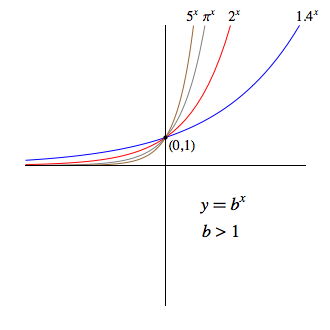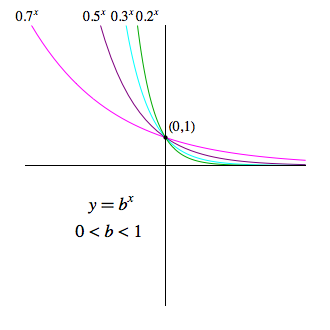## Exponential Functions

Recall that for any real number $b \gt 0$ and any real number $x$, the expression $b^x$ is defined and represents a unique, positive real number.

As such, for $b \gt 0$ and $b \neq 1$, we call the function $f(x) = b^x$ an exponential function, base $b$.

As we can see below, the nature of the graph for an exponential function depends largely on whether the base is greater than or less than one.The following additional properties of exponential functions of the form $f(x)=b^x$ are clearly evident from the graphs given above:

• The domain is the set of all reals, $\mathbb{R}$

• The range is the set of all positive reals, $(0,\infty)$

• The only intercept is $(0,1)$

• $y=0$ (i.e., the $x$-axis) is a horizontal asymptote

• The function increases over its entire domain if $b \gt 1$ and decreases over the same if $0 \lt b \lt 1$

• The function is one-to-one (i.e., invertible)

### The Natural Base, $e$

In mathematics, one is often concerned with invariants -- that is, things that don't change under a given action.

The study of calculus concerns itself with two primary "actions", differentiation and integration.

Without getting too far ahead of ourselves, the act of differentiation will take one function and produce another that gives the slope of the tangent line to the original function at any given $x$ value.

As will be discovered, excluding constant multiples of the same, there is only one function that doesn't change under the act of differentiation. This function turns out to be exponential -- but with a very strange base, namely:

$$e \approx 2.7182818$$

It may not seem so right now, but treating this irrational value as the most "natural" choice for a base of an exponential function turns out to be incredibly beneficial not only in the study of calculus but also in the mathematical analysis of many physical, sociological, and economic phenomena.

: Not only is e irrational, but it is also transcendental. That is to say, e is an irrational value that is not the root of any non-zero polynomial equation with rational coefficients.